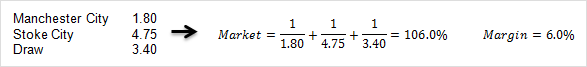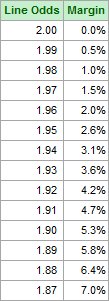# Bookmaker Margins

You are here:  Home >> Beginner’s Guide >> Betting Agencies >> Bookmaker Margins

### What are Bookmaker Margins?

When a bookmaker sets the odds for a market they first estimate the probability of each possible outcome. For example, in a market on a coin toss winner, the bookmaker will assume the probability of Team X winning is 50%. When using decimal odds, the fair odds for the wager equal the reciprocal of the bookmaker’s estimated probability, in this case 1 / 0.50 = 2.00. To fund their operation, the bookmaker adjusts these odds downward to create a profit margin for the market. For example, typical bookmaker odds on Team X winning a coin toss are 1.91. The difference between the published odds (1.91) and the fair odds (2.00) is the bookmaker margin.

The bookmaker margin is a measure of the bookmaker’s profit margin for an event and is a hidden transaction cost for punters. This profit is how bookmakers finance their services but bookmakers vary in the margins they apply. From a punter’s perspective, the lower the margin, the better. The difference between 1.90 and 1.92 line odds may not seem significant for a single wager, but when betting repeatedly this difference has a compounding effect. This can easily make the difference between winning and losing money.

Unfortunately there are conflicting definitions of bookmaker margin and a synonymous term vigorish. Even on Wikipedia the explanation of how to calculate vigorish depends on which article you visit. This website uses the definition of bookmaker margin (also referred to as the overround) as used in the Mathematics of bookmaking Wikipedia article and the definition of vigorish as used in the Vigorish Wikipedia article.

Below are calculation examples using two-outcome and three-outcome events.### Interpreting Bookmaker Margins

The margin measures the bookmaker’s profit if they were to receive wagers on each outcome in proportion to the odds. Suppose a bookmaker offers decimal odds a on outcome A and odds b on outcome B. If proportion b/(a+b) is wagered on outcome A and a/(a+b) is wagered on outcome B, then the bookmaker will receive the same profit regardless of the result.

 For example, recall that the bookmaker margin for the two-outcome event above was 3.5%. Suppose \$100,000 in total is wagered on the market, with:      (1.64/(1.64 + 2.35)) x \$100,000 = \$41,102.76 wagered on Oklahoma City and      (2.35/(1.64 + 2.35)) x \$100,000 = \$58,897.24 wagered on Denver. Depending on the outcome, the bookmaker will pay out one of the following two amounts:      If Oklahoma City wins: \$41,102.75 x 2.35 = \$96,591.48      If Denver wins: \$58,897.24 x 1.64 = \$96,591.48 The bookmaker accepts \$100,000 in wagers but only pays out \$96,591.48 to the winners. The profit margin is (\$100,000 – \$96,591.48)/\$96,591.48 = 3.5%, as calculated by the margin earlier. A bookmaker will rarely receive wagers that are perfectly in proportion to the odds, but over a countless number of events the bookmaker margin will equate to the average profit margin across all events. To provide perspective on how odds relate to margins, the table on the right compares equal line odds (bets with a 50% chance of winning) to their respective margins. Note that 2.00 odds equate to a margin of 1.00 (100%), where the bookmaker makes no profit on the market.### Bookmaker Margin Survey

Australia Sports Betting has conducted a survey of margins used by popular bookmakers.

### Combined Margins

For each market a “combined” margin can be calculated by sourcing the best available odds across multiple bookmakers. This combined margin represents the realisable margin that can be attained through holding a portfolio of bookmaker memberships and shopping around for the highest odds.

The combined margin is always equal to or lower than the lowest bookmaker’s margin. The greater the disparity between the odds of two or more bookmakers, the lower the combined margin.

For example, in the 2021 US Open men’s singles match between Djokovic and Brooskby, we observed the following head-to-head markets:

bet365:
Djokovic: 1.04
Brooskby: 13.00

PlayUp:
Djokovic: 1.01
Brooskby: 18.75

For this market bookmaker margin for bet365 is 3.85% while the margin for PlayUp is 4.34%.

If we combine the two markets by taking the highest available odds we get:
Djokovic: 1.04
Brooskby: 18.75

The combined margin for this market is 1/1.04 + 1/18.75 – 1 = 1.49%.

Combined margins are a crucial for beating bookmakers because they lowers the hurdle rate required to achieve a profit. The equivalent line odds for the 3.85% margin at bet365 is 1.926. To make a profit on 1.926 odds you would have to win more than 51.9% of the time. In contrast, the equivalent line odds for the 1.49% combined margin is 1.971. To make a profit on 1.971 odds you would only need to win more than 50.7% of the time.

Note that if the combined margin is a negative number then an arbitrage opportunity exists.

### Bookmaker Margin vs. Vigorish (Vig)

Another popular term, particularly in the United States, for measuring the bookmaker profit margin is vigorish. It is often referred to as vig, for short, or juice. In the context of this article the term vigorish refers to a different calculation to bookmaker margin. In short, the bookmaker margin is the bookmaker’s profit margin relative to the payouts to the winners, while vigorish refers to the profit margin relative to turnover, i.e. the initial wagers. Because the total payout is always less than the total turnover, the bookmaker margin is greater than vigorish for positive margins.

Referring to the previous NBA example:
The bookmaker margin is 1/1.64 + 1/2.35 – 1 = 3.53%
while vigorish is (1 – 1.64*2.35/(1.64 + 2.35)) = 3.41%

Recall that with this example, if \$41,102.76 is wagered on Oklahoma City and \$58,897.24 is wagered on Denver, the bookmaker accepts \$100,000 in wagers and pays out \$96,591.48 to the winners, regardless of the result. The bookmaker margin is (\$100,000 – \$96,591.48)/\$96,591.48 = 3.53%, while vigorish is (\$100,000 – \$96,591.48)/\$100,000 = 3.41%.

Both of these measures of bookmaker profit margin are valid, providing you understand what each represents. You can think of bookmaker margin as metres and vigorish as yards.

Note that if the bookmaker margin is 0% then vigorish is also 0%. If one is negative then so is the other.

For a markets with n outcomes the general formula for vigorish when using decimal odds is:Where odds is a set of odds(1), odds(2), odds(3)… odds(n).

You can convert back and forth between the bookmaker margin / overround and vigorish using the formulae below:

vig = bookmaker_margin / (1 + bookmaker_margin)

bookmaker_margin = vig / ( 1- vig)

### Margin Calculator

To better understand bookmaker margins and vigorish, check out our bookmaker margin / vigorish calculator.

 << Previous topic: Depositing & Withdrawing Funds Next topic: Multi Betting >>